## Monday, July 30, 2018

### Old Blue Eyes

(Click on the comic if you can't see the full image.)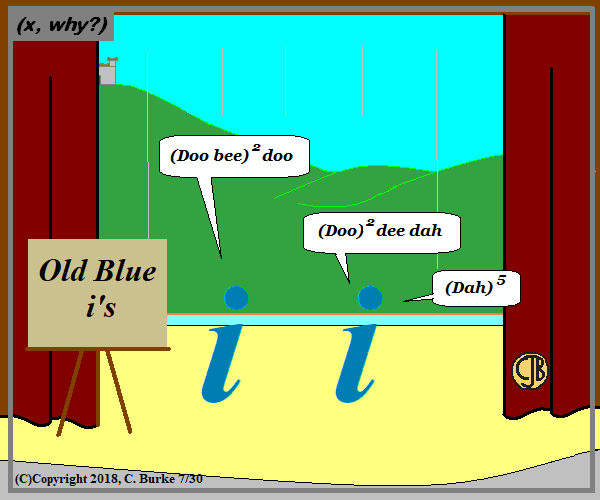Don't be the negative one here. That's his job.

I can't believe I haven't done this before. And there may be more of this to come.

Come back often for more funny math and geeky comics.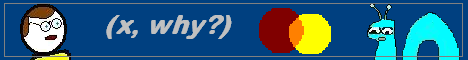## Saturday, July 28, 2018

### The Casebook of Sherlock Pi: The Noble Bachelor

(Click on the comic if you can't see the full image.)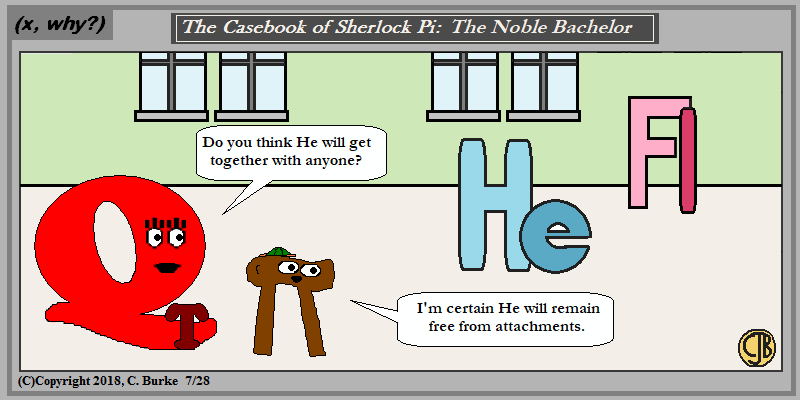He is happy just as He is. Say what you want, you won't get a reaction.

Come back often for more funny math and geeky comics.## Friday, July 27, 2018

### Togetherness

(Click on the comic if you can't see the full image.)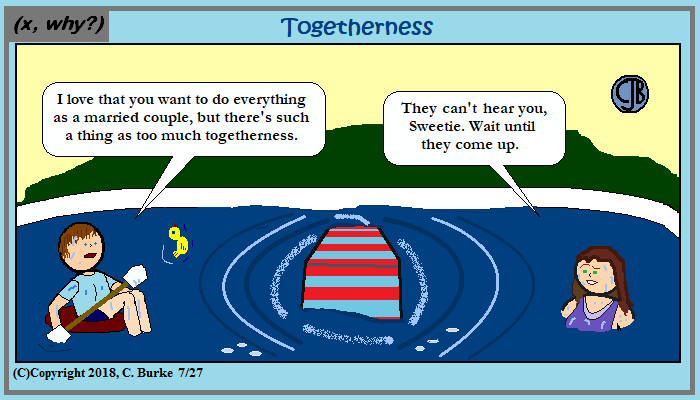Mr. & Mrs. Wayne will pop up any time now.

I wanted a Ken-and-Michele-are-back comic, and then I left them out of it.

I also left out the math -- probability that two people would capsize the chair was approximately 1.0.

I also figured that they would have gone out with Judy and Chuck first, but where's the math in that? Other than figuring the tip? (Chuck might be the least mature of the group, but I didn't want him to be a dweeb or anything.)

Come back often for more funny math and geeky comics.## Thursday, July 26, 2018

### Parallel vs. Perpendicular vs. Skew: Getting Shiggy edition

(Click on the comic if you can't see the full image.)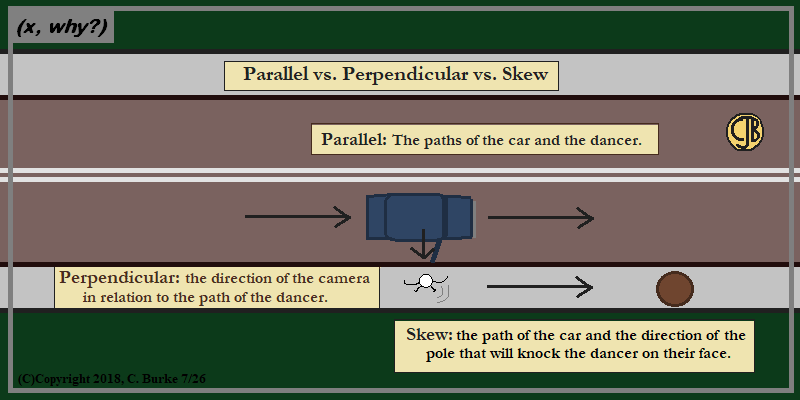It's only skew if the dancer *misses* the pole. But that wouldn't be as funny!
Don't try this at home. Or on the road.

Don't get ''shiggy'' with it.

Come back often for more funny math and geeky comics.## Wednesday, July 25, 2018

### (x, why?) Mini: 10 to the -6th Aggressions

(Click on the comic if you can't see the full image.)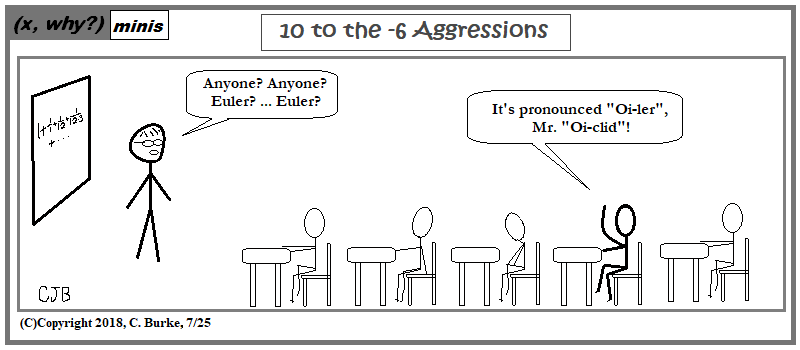I had a couple different ideas, but thise was the quicker one to do after my day off.

Come back often for more funny math and geeky comics.## Monday, July 23, 2018

### June 2018 Common Core Geometry Regents, Parts III and IV

The following are some of the multiple questions from the recent June 2018 New York State Common Core Geometry Regents exam.
The answers to Part I can be found here
The answers to Part II can be found here

### June 2018 Geometry, Part III

Each correct answer is worth up to 4 credits. Partial credit can be given. Work must be shown or explained.

32. Triangle ABC has vertices with coordinates A(-1,-1), B(4,0), and C(0,4). Prove that ABC is an isosceles triangle but not an equilateral triangle. [The use of the set of axes below is optional.]

If it is isosceles, then at least two legs have the same length. If it is not equilateral, then the third leg will have a different length.
Looking at the coordinates of the points, it should be obvious that AB and AC are congruent because you'll be using the same numbers in the calculations.
AB = SQRT ( (-1 - 4)2 + (-1 - 0)2 ) = SQRT(26)
AC = SQRT ( (-1 - 0)2 + (-1 - 4)2 ) = SQRT(26)
BC = SQRT ( (4 - 0)2 + (0 - 4)2 ) = SQRT(32)
There is no need to simplify because you're only looking for equality or inequality.
AB is the same length as AC but not the same as BC. Only two sides are congruent, so ABC is isosceles but not equilateral.

33. The map of a campground is shown below. Campsite C, first aid station F, and supply station S lie along a straight path. The path from the supply station to the tower, T, is perpendicular to the path from the supply station to the campsite. The length of path FS is 400 feet. The angle formed by path TF and path FS is 72°. The angle formed by path TC and path CS is 55°.

Determine and state, to the nearest foot, the distance from the campsite to the tower.

We can find the length of TS by using the tangent function with triangle TSF.
Once we know TS, we can use the sine function with triangle TSC to find the length of CT, the distance from the campsite to the tower.
Make sure your calculator is in Degree mode.

Tan 72 = x / 400
x = 400 * tan 72 = 1231.07...

Sin 55 = 1231.07 / y
y = 1231.07 / sin 55 = 1502.85897... = 1503 feet.
The distance from the campsite to the tower is 1503 feet.

Note that you could have skipped the intermediary skip and used (x / 400) in the last equation. Finding the length of TS was not required for the problem.

34. Shae has recently begun kickboxing and purchased training equipment as modeled in the diagram below. The total weight of the bag, pole, and unfilled base is 270 pounds. The cylindrical base is 18 inches tall with a diameter of 20 inches. The dry sand used to fill the base weighs 95.46 lbs per cubic foot.

To the nearest pound, determine and state the total weight of the training equipment if the base is filled to 85% of its capacity.

Find the Volume of the Base in cubic feet (not cubic inches). Multiply that by .85 to find 85% of the Volume. Multiply that by 95.46 to find the weight of the sand in the base. Then add 270 pounds for the bag, pole and unfilled base.
Remember that the radius is half the diameter: 20 / 2 = 10 inches, which is 10/12 of a foot. The height is 18 inches, which is 18/12 feet.
V = pi * r2 * h = (3.141592...)(10/12)2 * 18/12 = 3.27249
.85V = .85 * 3.27249 = 2.7816 cubic feet.
The weight of the sand = 2.7816 * 95.46 = 265.53 pounds.
Total weight = 265.53 + 270 = 535.53 or 536 pounds.

End of Part III

### Part IV

A correct answer is worth up to 6 credits. Partial credit can be given. Work must be shown or explained.

35. Parallelogram ABCD, BF ⊥ AFD, and DE ⊥ BEC.

Prove: BEDF is a rectangle

BEDF is a rectangle if it is a parallelogram as has a right angle. You need not prove the length of the opposite sides are congruent.
1. ABCD is a parallelogram, BF ⊥ AFD, DE ⊥ BEC: Given
2. BC || AD: Opposite sides of a parallelogram are parallel.
3. BE || DE: Parts of parallel lines are parallel.
4. BF || DE: Two lines that are perpendicular to the same line are parallel.
5. BEDF is a parallelogram: A quadrilateral with two pairs of parallel sides is a parallelogram.
6. Angle DEB is a right angle: Perpendicular lines form right angles.
Note: Line 6 wasn't actually *Given*, even though the boxes for right angles are shown in the diagram. Whatever reason you give, the fact that it is a right angle is important and must be stated!
7. BEDF is a rectangle: A parallelogram with a right angles is a rectangle.

End of Part IV

How did you do?

## Saturday, July 21, 2018

### Trigonometry Jones and the i of Vector

(Click on the comic if you can't see the full image.)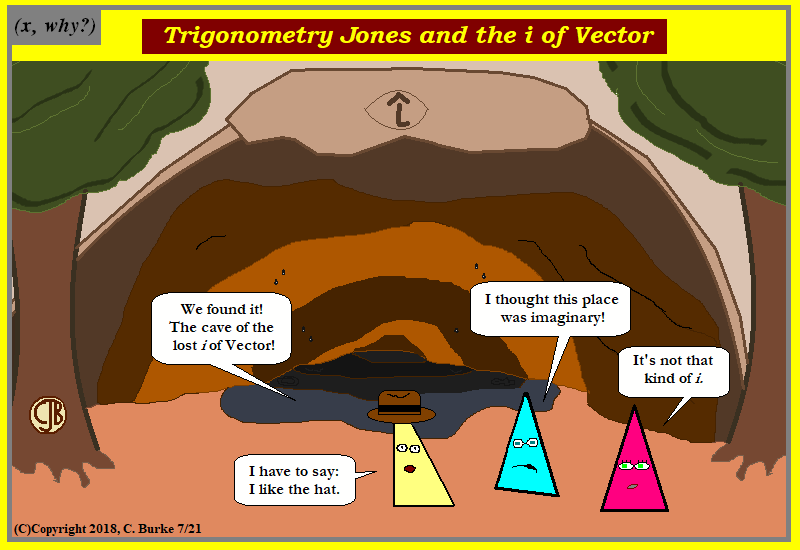It uses the Right-Hand Rule because everyone lose that Vector's left-hand was lost and became a powerful artifact!

Come back often for more funny math and geeky comics.## Friday, July 20, 2018

### (x, why?) Mini: Altitude

(Click on the comic if you can't see the full image.)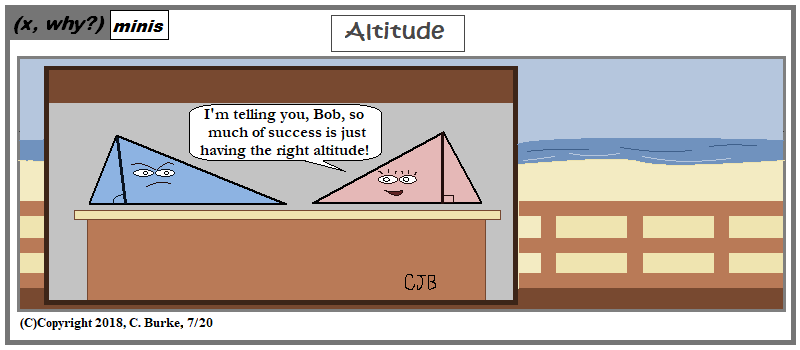For instance, finding the area, altitude is key!

Come back often for more funny math and geeky comics.## Wednesday, July 18, 2018

### The Young Pioneers

(Click on the comic if you can't see the full image.)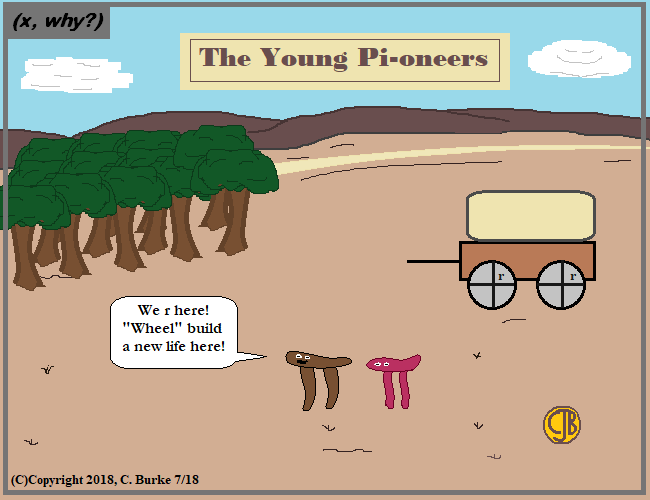Episode 2: Building a Log Cabin

Come back often for more funny math and geeky comics.## Tuesday, July 17, 2018

### June 2018 Common Core Geometry Regents, Part II

The following are some of the multiple questions from the recent June 2018 New York State Common Core Geometry Regents exam.
The answers to Part I can be found here

### June 2018 Geometry, Part II

Each correct answer is worth up to 2 credits. Partial credit can be given. Work must be shown or explained.

25. Triangle A'B'C' is the image of triangle ABC after a translation of 2 units to the right and 3 units up. Is triangle ABC congruent to triangle A'B'C'? Explain why

Yes, because a translation is a rigid motion, which preserves shape and size.
The corresponing angles are congruent, and the corresponding sides are congruent, so the triangles are congruent.

26. Triangle ABC and point D(1,2) are graphed on the set of axes below.

See image below.

Graph and label triangle A'B'C', the image of triangle ABC, after a dilation of scale factor 2 centered at point D.

See image below. No explanation is needed. Just the graph, with labels.
Each image is twice as far away from D as the original point was.
To get from D to A, go down 1 unit and 3 left. To get from A to A', do this again. A' is (-5, 0)
To get from D to B, go up 3 units and 1 left. To get from B to B', do this again. B' is (-1, 8)
To get from D to C, go down 3 units and 3 right. To get from C to C', do this again. C' is (7, -4)

27. Quadrilaterals BIKE and GOLF are graphed on the set of axes below.

Describe a sequence of transformations that maps quadrilateral BIKE onto quadrilateral GOLF.

Notice that letters of BIKE go counterclockwise and GOLF go clockwise. A simple translation will not be good enough. A translation of T5,8 will map BIKE over GOLF; however, B doesn't map to G, and K doesn't map to L.
You need to include a reflection in your transformations. The directions ask for a sequence.
There are many possibilities, but the simplest (to me) would be a reflection over the y-axis followed by a translation of 5 units up.

28. In the diagram below, secants RST and RQP, drawn from point R, intersect circle O at S, T, Q, and P.

If RS = 6, ST = 4, and RP = 15, what is the length of RQ?

(RS)(RT) = (RQ)(RP)
(6)(6 + 4) = (RQ)(15)
60 = 15(RQ)
RQ = 4.

29. Using a compass and straightedge, construct the median to side AC in ABC below.
[Leave all construction marks.]

See image.
To construct a median, you need to find the midpoint of AC. You can find the midpoint by constructing a perpendicular bisector of AC. Label the midpoint D. Then use the straightedge to draw BD.

30.
Skye says that the two triangles below are congruent. Margaret says that the two triangles are similar.
Are Skye and Margaret both correct? Explain why.

Skye and Margaret are both correct. Using the Pythagorean Theorem, 52 + 122 = 169, which is 132, so the length of the hypotenuse of the first triangle is 13. By the Hypotenuse-Leg Theorem, the two triangles are congruent.
If the triangles are congruent, then their corresponding angles are congruent, and that makes them similar triangles as well.

31. Randy’s basketball is in the shape of a sphere with a maximum circumference of 29.5 inches. Determine and state the volume of the basketball, to the nearest cubic inch.

The circumference is 2*pi*r = 29.5
So the radius, r = 29.5 / (2 * pi) = 4.695...
Volume of a sphere = (4/3) * pi * r3 = (4/3) * pi * (4.695)3 = 433.506...
To the nearest cubic inch, the Volume is 434 cubic inches.

End of Part II

How did you do?

## Monday, July 16, 2018

### June 2018 Common Core Geometry Regents, Part I (multiple choice)

The following are some of the multiple questions from the recent June 2018 New York State Common Core Geometry Regents exam.

### June 2018 Geometry, Part I

Each correct answer is worth up to 2 credits. No partial credit. Work need not be shown.

1. After a counterclockwise rotation about point X, scalene triangle ABC maps onto triangle RST, as shown in the diagram below.

Which statement must be true?

A rigid motion, such as a rotation, does not change size, so the corresponding angles and sides remain the same.
Angle A corresponds to Angle R. Angle B corresponds to Angle S. Angle C corresponds to Angle T.
Choice 2 does not have corresponding angles. Choices 3 and 4 do not have corresponding sides.ity symbol.

2. In the diagram below, AB || DEF, AE and BD intersect at C, m∠B = 43°, and m∠CEF = 152°.

Which statement is true?

Because of alternate interior angles, angle D = 43 degrees. Angle CED is supplementary to 152 degrees, so it must be 180 - 152 = 28 degrees.
By the Exterior Angle Theorem, Angle ACD must be the sum of the two remote angles, D and CED. So 43 + 28 = 71 degrees.

3. In the diagram below, line m is parallel to line n. Figure 2 is the image of Figure 1 after a reflection over line m. Figure 3 is the image of Figure 2 after a reflection over line n.

Which single transformation would carry Figure 1 onto Figure 3?

A translation will move Figure 1 directly to Figure 3. Reflecting the figure switched the orientation, but reflecting it a second time switched it back to its original orientation.
A dilation would have increased its size. A rotation would have changed its orientation, as would a single reflection.

4. In the diagram below, AF and DB intersect at C, and AD and FBE are drawn such that m∠D = 65°, m∠CBE = 115°, DC = 7.2, AC = 9.6, and FC = 21.6.

What is the length of CB?

If angle DBE = 115 degrees, then angle DBF = 65 degrees. This makes angles DBF and D congruent. Angles ACD and BCF are congruent because they are vertical angles. Therefore, the two triangles are similar. (Of course, if these triangles weren't similar to begin with, this would've been a much harder problem to deal with!)
That means that the corresponding sides are proportional.
7.2 / 9.6 = x / 21.6
9.6x = (7.2)(21.6)
x = (7.2)(21.6)/9.6 = 16.2

5. Given square RSTV, where RS = 9 cm. If square RSTV is dilated by a scale factor of 3 about a given center, what is the perimeter, in centimeters, of the image of RSTV after the dilation?

If RS = 9, then the perimeter is 9 * 4 = 36. If the square is dilated by a factor of 3, then the perimeter will be 3 times as much. So 36 * 3 = 108.
Alternatively, 9 * 3 = 27 cm as the length of the side of the image, and 27 * 4 = 108.

6. In right triangle ABC, hypotenuse AB has a length of 26 cm, and side BC has a length of 17.6 cm. What is the measure of angle B, to the nearest degree?

cos B = 17.6 / 26
B = cos-1 (17.6/26) = 47.396... or 47 degrees.

7. The greenhouse pictured below can be modeled as a rectangular prism with a half-cylinder on top. The rectangular prism is 20 feet wide, 12 feet high, and 45 feet long. The half-cylinder has a diameter of 20 feet.

To the nearest cubic foot, what is the volume of the greenhouse?

The Volume of the rectangular prism is Length x Width x Height. The volume of the half-cylinder is 1/2 pi x radius2 x height. Don't confuse to two things that are labeled "height".
Vp = (12)(20)(45) = 10800
Vc = (1/2)(10)2(pi)(45) = 7068.583...
For a total of approximately 17,869

8. In a right triangle, the acute angles have the relationship sin (2x + 4) = cos (46). What is the value of x?

In a right triangle, the relationship between sin and cos of the acute angles is as follows:

sin (O) = cos (90 - O)
So if sin(2x + 4) = cos(46) then 2x + 4 = 90 - 46
2x + 4 = 44
2x = 40
x = 20

9. In the diagram below, AB||DFC, EDA||CBG, and EFB and AG are drawn.

Which statement is always true?

Answer: (4) Triangle DEF ~ Triangle AEB
Because AB || EDF then Angle A = Angle EDF, and angle E is congruent to itself by the Reflexive Property. By AA, triangles DEF and AEB are similar.
Of the incorrect answers, choice (1) would have been correct had it said "similar to" and not "congruent to", because of alternate interior angles and vertical angles. However, there is no indication that F is the midpoint of EB or CD, or that BC is congruent to DE.

10. The base of a pyramid is a rectangle with a width of 4.6 cm and a length of 9 cm. What is the height, in centimeters, of the pyramid if its volume is 82.8 cm3?

The formula for the Volume of a rectangular-based pyramid is V = 1/3 L * W * H.
So 82.8 = (1/3) * (4.6) * (9) * H.
And H = 82.8 / ((1/3) * (4.6) * (9))
H = 6 cm.

11. In the diagram below of right triangle AED, BC || DE.

Which statement is always true?

Short hypotenuse / Long hoptenuse = Short altitude / Long altitude
The incorrect choices:
(1) Short base / Long base =/= Long altitude / Short altitude -- incorrect order
(3) Short base / *segment* of long base =/= Short altitude / Long altitude -- not corresponding parts
(4) Long altitude / Short altitude =/= *segment* of long hypotenuse / Short hypotenuse -- not corresponding parts

12. What is an equation of the line that passes through the point (6,8) and is perpendicular to a line with equation y = 3/2 x + 5?

Answer: (2) y - 8 = - 2/3 (x - 6)
Perpendicular means that the slope of the new line is -2/3, so eliminate choices (1) and (3), which are parallel.
Point-slope form is y - y0 = m(x - x0). Note the signs.
Plug in 6 for x0 and 8 for y0

13. The diagram below shows parallelogram ABCD with diagonals and intersecting at E.

What additional information is sufficient to prove that parallelogram ABCD is also a rhombus?

Answer: (4) AC is perpendicular to BD
The diagonals of a rhombus are perpendicular to each other.
Choices (1), (2), and (3) are true for all parallelograms, not just rhombuses.

14. Directed line segment DE has endpoints D(-4,-2) and E(1,8). Point F divides such that DF:FE is 2:3. What are the coordinates of F?

2 + 3 = 5, so point F is 2/5 of the way from D to E.
1 - (-4) = 5, and (2/5)(5) = 2. Add 2 to -4 and you get -2, which must be the x-coordinate for F. So Choice (1).
8 - (-2) = 10, and (2/5)(10) = 4. Add 4 to -2 and you get 2, which must be the y-coordinate for F. Still Choice (1).
Notice that point F must be on the line BETWEEN D and E, so Choice (4) makes no sense at all.

15. Triangle DAN is graphed on the set of axes below. The vertices of DAN have coordinates D(-6,-1), A(6,3), and N(-3,10).

What is the area of triangle DAN?

Reason this one out. It's a multiple-choice question, so you don't have to go the long way.
You might notice that the slope of DA is 1/3. That means that the altitude of the triangle can be found by starting at N(-3, 10) and finding points that are 3 down and 1 to the right, because the perpendicular slope is -3. This gives you the point (0, 1). Call it P.
You could then find the length of AD and NP, and then use those vales as base and height in the formula (1/2) b h.
HOWEVER, there is another way. Make a big rectangle around the triangle, so that D, A, and N are each touching a side of the rectangle.
This rectangle with have dimensions 11 X 12, or 132 square units.
Now remove the "negative" space -- that is, the three triangles outside of DAN.
First thing you will realize is that all the bases and heights are whole numbers, so their areas are rational. That means that choices (3) and (4) cannot be correct.
At this point, knowing how the Regents operates, you can be pretty sure that the answer is 60, and that 120 is there in case you forget to multiply by 1/2, a common error.

Doing it this way, you have 132 - (1/2)(11)(3) - (1/2)(9)(7) - (1/2)(12)(4) = 60.

The long way: the length of AD is the square root of ((-6 - 6)2 + (-1 - 3)2)) = SQRT(144 + 16) = SQRT(160)
The length of NP is the square root of ((-3 - 0)2 + (10 - 1)2)) = SQRT(9 + 81) = SQRT(90)
A = (1/2) * SQRT(160) * SQRT(90) = (1/2) * SQRT(14,440) = (1/2)(120) = 60.

16. Triangle ABC, with vertices at A(0,0), B(3,5), and C(0,5), is graphed on the set of axes shown below.

Which figure is formed when ABC is rotated continuously about BC?

When you rotate the triangle, you will get a cone. The axis BC that it is rotated around, will be the altitude of the cone. The base of the triangle will become the radius of the cone.
So the height is 3, the radius is 5 and the diameter is 10. Choice (3).
Note that Choices (1) and (2) are rotated about the wrong line.

17. In the diagram below of circle O, chords AB and CD intersect at E.
If mAC = 72° and m∠AEC = 58°, how many degrees are in mDB ?

58 is the average of 72 and mDB.
58 = (72 + x)/2
2(58) = 72 + x
116 - 72 = x
44 = x.
Alternatively, 72 - 58 = 14, and 58 - 14 = 44.

18. In triangle SRK below, medians SC, KE, and RL intersect at M.
Which statement must always be true?

The three medians of a triangle meet at a centroid, which is point M. The distance from the angle to the centroid is ALWAYS twice as much as from the centroid to the midpoint of the opposite side. That always means that the distance from the centroid to the midpoint is one-third the length of the entire median. So 3(MC) = SC is always true.
Incorrect choices: (2) MC is 1/3 of SC, not 1/3 of SM; (3) RM is 2 times ML, not MC, which is unrelated to RM; (4) SM and KM could be the same size but there is no reason that they must be.

19. MThe regular polygon below is rotated about its center.
Which angle of rotation will carry the figure onto itself?

One full rotation is 360 degrees. A regular pentagon will carry onto itself every 1/5th of a rotation.
360 / 5 = 72 degrees. So this will happen at any multiple of 72 degrees.
72 * 3 = 216.

Incorrect choices: (1) 60 degrees is for a hexagon; (2) 108 degrees is the measure of one interior angle of a regular pentagon; (4) 540 degrees is the total number of degrees of the interior angles.

20. What is an equation of circle O shown in the graph below?

Answer: (2) x2 - 10x + y2 - 4x = -13
The standard form for a circle is (x - h)2 + (y - k)2 = r2, where (h,k) is the center.
The center is (5,2) and the radius is 4, so (x - 5)2 + (y - 2)2 = 42.
The choices are not in standard form, so you have to square the binomials.
x2 - 10x + 25 + y2 - 4x + 4 = 16
x2 - 10x + y2 - 4x = 16 - 25 - 4
x2 - 10x + y2 - 4x = -13

21. In the diagram below of PQR, ST is drawn parallel to PR, PS = 2, SQ = 5, and TR = 5.

What is the length of QR?

The ratio between the side lengths is the same. So if PS = 2 and RT = 5, then QR is 2.5 times the length of PQ. And QR is 2.5 times as long as QP, so 2.5 * 7 = 17.5
Or you can write a proportion to find QT.
5 / 7 = x / (x + 5)
7x = 5(x + 5)
7x = 5x + 25
2x = 25
x = 12.5
If QT = 12.5 and TR = 5, then QR = 12.5 + 5 = 17.5

22. The diagram below shows circle O with radii OA and OB. The measure of angle AOB is 120°, and the length of a radius is 6 inches.

Which expression represents the length of arc AB, in inches?

The length of an arc of the circle is the Circumference (2πr) times the fraction of the central angle divided by 360.
(120/360)(2π)(6) = (120/360)(12π) = (1/3)(12π)

23. Line segment CD is the altitude drawn to hypotenuse EF in right triangle ECF. If EC = 10 and EF = 24, then, to the nearest tenth, ED is

If it helps, draw a diagram.

ED is the shorter leg of right triangle EDC, and EC is the shorter leg of triangle ECF.
EC is the hypotenuse of right triangle EDC, and EF is the hypotenuse of triangle ECF.
Write a proportion:
x / 10 = 10 / 24
24x = (10)(10)
x = 100 / 24 = 4.2

If you draw a diagram, you can see that ED had to be shorter than EC, so choices (3) and (4) make no sense. Eliminate them.

24. Line MN is dilated by a scale factor of 2 centered at the point (0,6). If is MN represented by y = -3x + 6, which equation can represent M'N', the image of MN?

Answer: (2) y = -3x + 6
A dilation would not change the slope of a line, so eliminate Choices (3) and (4).
A dilation would only change the y-intercept UNLESS the line goes through the center of dilation -- that is, if the center of dilation is a point on the line. In that case, the line remains unchanged because the image of every point will still fall on the same line.
Check for this: 6 = -3(0) + 6
6 = 6, which is true, so the center of dilation is on the line, and the image is the same line.

End of Part I

How did you do?

## Saturday, July 14, 2018

### The Casebook of Sherlock Pi: Orange Pips Five

(Click on the comic if you can't see the full image.)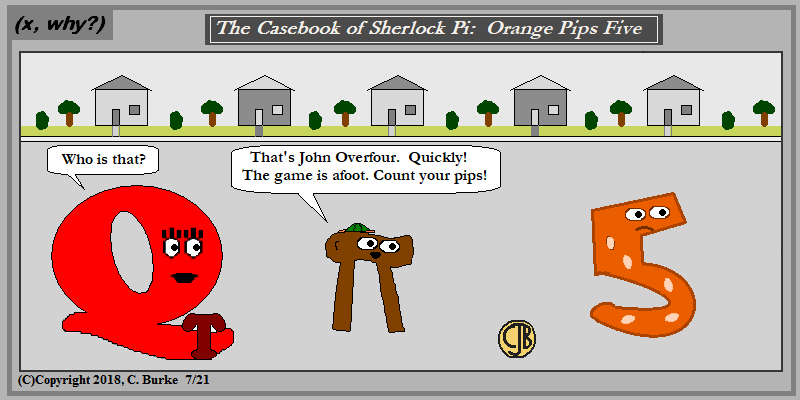With all those pips, you'll be glad it's night.

Come back often for more funny math and geeky comics.## Friday, July 13, 2018

### Not That Long

(Click on the comic if you can't see the full image.)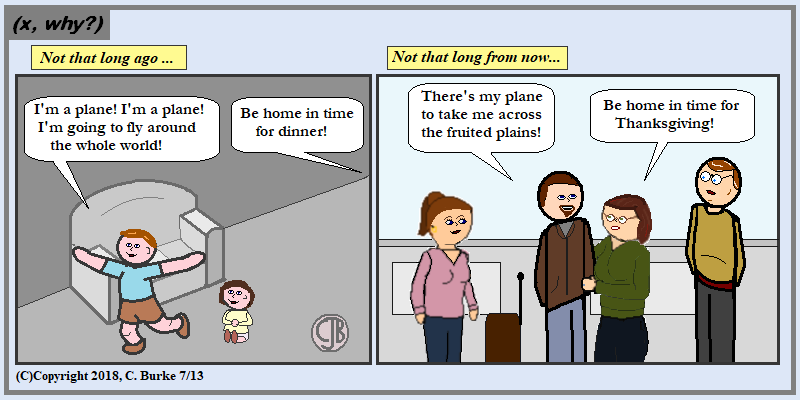Congrats, and good luck, to my son who is flying off across the country to start his real career job.

It wasn't that long ago ...

Come back often for more funny math and geeky comics.## Thursday, July 12, 2018

### June 2018 Common Core Algebra I Regents, Parts III and IV

The following are some of the multiple questions from the recent June 2018 New York State Common Core Algebra I Regents exam.
The answers to Part I can be found here
The answers to Part II can be found here

### June 2018 Algebra I, Part III

Each correct answer is worth up to 4 credits. Partial credit can be given. Work must be shown or explained.

33. A population of rabbits in a lab, p(x), can be modeled by the function p(x) = 20(1.014)x, where x represents the number of days since the population was first counted.
Explain what 20 and 1.014 represent in the context of the problem.
Determine, to the nearest tenth, the average rate of change from day 50 to day 100.

In the context of the problem, 20 is the initial population of the rabbits and 1.014 is the rate of growth of the rabbit population of 1.4% per day.
1.014 = 101.4%, which is 1.4% increase.

To find the average rate of change from day 50 to day 100, find p(50) and p(100). Subtract them and divide by 100 - 50 = 50.
p(50) = 20(1.014)50 = 40.08...
p(100) = 20(1.014)100 = 80.32...
(80.32 - 40.08) / (100 - 50) = 40.24/50 = 0.8048. which is approximately 0.8 to the nearest tenth.
The rate of change is 0.8.

34. There are two parking garages in Beacon Falls. Garage A charges \$7.00 to park for the first 2 hours, and each additional hour costs \$3.00. Garage B charges \$3.25 per hour to park.
When a person parks for at least 2 hours, write equations to model the cost of parking for a total of x hours in Garage A and Garage B.
Determine algebraically the number of hours when the cost of parking at both garages will be the same.

A: y = 3.00(x - 2) + 7.00 (\$7 for the first 2 hours. Those 2 hours are subtracted from x)
B: y = 3.25x (All hours are the same.)

3.25x = 3.00(x - 2) + 7.00
3.25x = 3.00x - 6.00 + 7.00
.25x = 1.00
x = 4
They will cost the same after 4 hours.

35. On the set of axes below, graph the following system of inequalities:

2y + 3x < 14
4x - y < 2

Determine if the point (1,2) is in the solution set. Explain your answer.

Note that the y term is first in the first inequality.
If you are rewriting these into slope-intercept form to put them into your calculator, be mindful of the inequality symbol.

 2y + 3x < 14 2y < -3x + 14 y < (-3/2)x + 7 negative slope, solid line, shaded below 4x - y < 2 - y < -4x + 2 y > 4x - 2 positive slope, broken line, shaded above (left)

See image below.
The point (1, 2) is NOT in the solution set because it is on a broken line, which is not part of the solution.

36. The percentage of students scoring 85 or better on a mathematics final exam and an English final exam during a recent school year for seven schools is shown in the table below.

 Percentage of StudentsScoring 85 or Better Mathematics, x English, y 27 46 12 28 13 45 10 34 30 56 45 67 20 42

Write the linear regression equation for these data, rounding all values to the nearest hundredth.
State the correlation coefficient of the linear regression equation, to the nearest hundredth. Explain the meaning of this value in the context of these data.

Put all the data into the first two lists on your graphing calculator. Make sure you have DiagnosticsOn (so you'll get the correlation coefficient), and run a linear regression. Round all values to TWO decimals (nearest hundredth).
You will get the equation y = .96x + 23.95
The correlation coefficient is r = .92.
In the context of these data, there is a strong positive correlation between the percentage of students scoring over 85 in Mathematics and English. As one increases, the other increases.

End of Part III

### June 2018 Algebra I, Part IV

A correct answer is worth up to 6 credits. Partial credit can be given. Work must be shown or explained.

37. Dylan has a bank that sorts coins as they are dropped into it. A panel on the front displays the total number of coins inside as well as the total value of these coins. The panel shows 90 coins with a value of \$17.55 inside of the bank.
If Dylan only collects dimes and quarters, write a system of equations in two variables or an equation in one variable that could be used to model this situation.
Using your equation or system of equations, algebraically determine the number of quarters Dylan has in his bank.
Dylan’s mom told him that she would replace each one of his dimes with a quarter. If he uses all of his coins, determine if Dylan would then have enough money to buy a game priced at \$20.98 if he must also pay an 8% sales tax. Justify your answer.

Let D be the number of dimes and Q be the number of quarters.
The value of the dimes will be .10D and the value of the quarters will be .25Q.
The equations you need to write to model this situation are:

D + Q = 90
.10D + .25Q = 17.55

To find the number of quarters, solve the system of equations.

D + Q = 90
.10D + .25Q = 17.55
-------------------
-.10D - .10Q = -9.0
.10D + .25Q = 17.55
-------------------
.15Q = 8.55
Q = 57
Dylan has 57 quarters in his bank.
Check:
D + 57 = 90, D = 90 - 57 = 33
.10(33) + .25(57) = 3.30 + 14.25 = 17.55 (check)

If his mom replaces all the dimes with quarters, then Dylan has 90 quarters, which are worth 90*.25= \$22.50
The game costs 20.98 + 20.98(0.08) = 22.6584 or \$22.66.
Dylan doesn't have enough money. He will be 16 cents short. (or \$.16, but NOT ".16 cents")
You could also multiply 20.98 times 1.08, which is the price plus 8%.

End of Part IV

How did you do?

## Wednesday, July 11, 2018

### June 2018 Common Core Algebra I Regents, Part II

The following are some of the multiple questions from the recent June 2018 New York State Common Core Algebra I Regents exam.
The answers to Part I can be found here

### June 2018 Algebra I, Part II

Each correct answer is worth up to 2 credits. Partial credit can be given. Work must be shown or explained.

25. Graph f(x) = SQRT(x+2) over the domain -2 < x < 7.

See graph below. The domain has two closed endpoints. No arrows. You only needed to plot the integer points
Note that this is an often-repeated question, with the graph translated.

26. Caleb claims that the ordered pairs shown in the table below are from a nonlinear function.

 x f(x) 0 2 1 4 2 8 3 16
State if Caleb is correct. Explain your reasoning.

Caleb is correct. The table does not have a constant rate of change so it cannot be linear.
That is all that is required. Everything below is extra information. Note that if you make a mistake with anything that follows you could lose a point.
Proof: (4 - 2)/(1 - 0) = 2/1 = 2; (8 - 4)/(2 - 1) = 4/1 = 4

In fact, this is an exponential function because it has a common ratio:
Proof: 4/2 = 2; 8/4 = 2; 16/8 = 2.

27. Solve for x to the nearest tenth: x2 + x - 5 = 0.

You can solve this using the Quadratic Formula or by Completing the Square. To complete the square would involve fractions (1/2 and 1/4), so let's use the Quadratic Formula. See image below.

Since the question did not say "calculate" or "algebraically", you also could have done this graphically. You could use your graphing calculator to graph the equation, and then use the Zeroes function. You would have to state this in some manner to justify your answer, because simply listing x = 1.8 or x = -2.8 as the answer is only worth 1 point.

28. The graph of the function p(x) is represented below. On the same set of axes, sketch the function p(x + 2).

p(x + 2) shifts p(x) two units to the left.
For example, when x = 0, p(0 + 2) = p(2) = 2. So (0, 2) is a point on the graph. See image below.

29. When an apple is dropped from a tower 256 feet high, the function h(t) = - 16t2 + 256 models the height of the apple, in feet, after t seconds. Determine, algebraically, the number of seconds it takes the apple to hit the ground.

Set the equation equal to 0 and then use inverse operations. Discard the negative square root.

-16t2 + 256 = 0
-16t2 = -256
t2 = 16
t = 4 seconds.

30. Solve the equation below algebraically for the exact value of x.

6 - (2/3) (x + 5) = 4x

Answer: You can get rid of the fraction by multiplying the entire equation by 3.

6 - (2/3) (x + 5) = 4x
(3)[6 - (2/3) (x + 5)] = [4x](3)
18 - 2(x + 5) = 12x
18 - 2x - 10 = 12x
8 - 2x = 12x
8 = 14x
x = 8/14 or x = 4/7

Since the wanted an exact value, leave the answer in fraction form because it's a repeating decimal. DO NOT ROUND.

31. Is the product of SQRT(16) and 4/7 rational or irrational? Explain your reasoning.

The product is rational because the square of 16 is 4, which is rational, and 4/7 is rational. The product of two rational numbers is always rational.
In this case, the product is 16/7, which is a ratio of two integers.

32. On the set of axes below, graph the piecewise function:

To graph this piecewise function, you need to graph f(x) = -1/2x, but only for the domain x < 2. The "Less than" sign indicates that 2 is NOT part of the domain, so f(2) = -1/2(2) = -1 is a BOUNDARY. In other words, you must graph this point, but with an open circle. Do not fill it in. Have an arrow on the left side only.
For the rest of the graph, for the domain x > 2, graph f(x) = x. That is (2, 2), (3, 3), (4, 4), etc. Closed endpoint on the left, arrow on the right.
See graph below.

End of Part II

How did you do?

## Monday, July 09, 2018

### (x, why?) Mini: Meet the ELs

(Click on the comic if you can't see the full image.)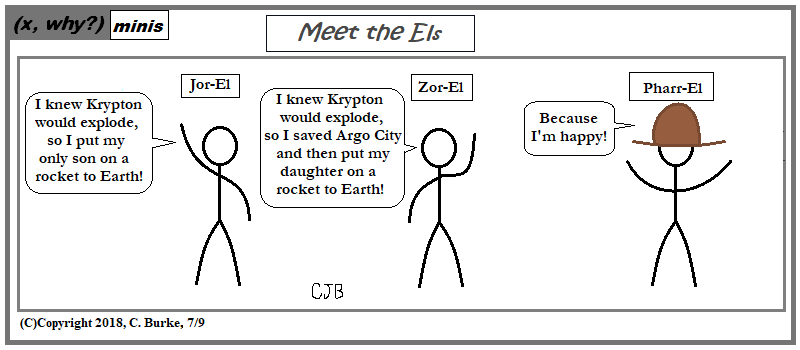And so only two rockets made it to Earth ...

Come back often for more funny math and geeky comics.